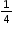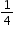# MA.3.FR.1.1

Represent and interpret unit fractions in the form 1/n as the quantity formed by one part when a whole is partitioned into n equal parts.

### Examplescan be represented asof a pie (parts of a shape), as 1 out of 4 trees (parts of a set) or ason the number line.

### Clarifications

Clarification 1: This benchmark emphasizes conceptual understanding through the use of manipulatives or visual models.
Clarification 2: Instruction focuses on representing a unit fraction as part of a whole, part of a set, a point on a number line, a visual model or in fractional notation.

Clarification 3: Denominators are limited to 2, 3, 4, 5, 6, 8, 10 and 12.

General Information
Subject Area: Mathematics (B.E.S.T.)
Strand: Fractions
Status: State Board Approved

## Related Courses

This benchmark is part of these courses.
5012050: Grade Three Mathematics (Specifically in versions: 2014 - 2015, 2015 - 2022, 2022 and beyond (current))
7712040: Access Mathematics Grade 3 (Specifically in versions: 2014 - 2015, 2015 - 2018, 2018 - 2022, 2022 and beyond (current))
5012055: Grade 3 Accelerated Mathematics (Specifically in versions: 2019 - 2022, 2022 and beyond (current))
5012015: Foundational Skills in Mathematics 3-5 (Specifically in versions: 2019 - 2022, 2022 and beyond (current))

## Related Access Points

Alternate version of this benchmark for students with significant cognitive disabilities.
MA.3.FR.1.AP.1: Explore unit fractions in the form 1/n as the quantity formed by one part when a whole is partitioned into n equal parts. Denominators are limited to 2, 3 and 4.

## Related Resources

Vetted resources educators can use to teach the concepts and skills in this benchmark.

## Educational Game

Flower Power: An Ordering of Rational Numbers Game:

This is a fun and interactive game that helps students practice ordering rational numbers, including decimals, fractions, and percents. You are planting and harvesting flowers for cash. Allow the bee to pollinate, and you can multiply your crops and cash rewards!

Type: Educational Game

## Formative Assessments

Unit Fractions:

Students divide a hexagon into two, three, and six equal parts and write the unit fraction representing each part.

Type: Formative Assessment

Two Equal Parts:

Students divide figures into two parts, each having the same area, and write the unit fraction representing each part.

Type: Formative Assessment

Which Shows One Third?:

Students are shown three circles and asked to select the one that correctly shows one third shaded and explain why the other two do not.

Type: Formative Assessment

What Does One Fifth Mean?:

Students are shown the fraction one fifth and asked to describe what it means.

Type: Formative Assessment

Halves of an Irregular Polygon:

Students partition an irregular hexagon into two equal parts and describe each part using a unit fraction.

Type: Formative Assessment

Four Parts of the Whole:

Students partition a rectangle into four equal parts and describe each part using a fraction.

Type: Formative Assessment

## Image/Photograph

Clipart ETC Fractions:

Illustrations that can be used for teaching and demonstrating fractions. Fractional representations are modeled in wedges of circles ("pieces of pie") and parts of polygons. There are also clipart images of numerical fractions, both proper and improper, from halves to twelfths. Fraction charts and fraction strips found in this collection can be used as manipulatives and are ready to print for classroom use.

Type: Image/Photograph

## Lesson Plans

Mystery Strips - Fractional Parts of the Whole:

This problem-solving lesson has students working together in groups to discover that fractions are made up of equal parts of one-whole. In this lesson, students are exposed to equivalent fractions as well as challenged to work beyond unit fractions to discover the fractional part of one-whole that the "mystery strips" represent. This highly engaging lesson takes into account all levels of learners and will challenge even your most advanced students.

Type: Lesson Plan

Fraction Action!:

This lesson will help students understand that fractions are parts of a whole. The lesson introduces fractional parts using familiar manipulatives.

Type: Lesson Plan

Discovering Fractions:

In this lesson students will make initial discoveries about fractions. Students will work together to explain and record the discoveries they make while using manipulatives to explore fractions.

Type: Lesson Plan

Fractions Meet Pattern Blocks:

Students will identify the fractional parts of a whole using pattern blocks.

Type: Lesson Plan

Symmetrical Solutions:

Students will use paper cutout and geoboards to find and create lines of symmetry. Students will have the opportunity to work with a partner and independently.

Type: Lesson Plan

Fraction Folding - Part 2:

Students will use foldables to create and name fractions. Students will sing a song to learn the terms numerator and denominator. Students will identify how many unit fractions compose a fraction.

Type: Lesson Plan

## Original Student Tutorials

Finding Fractions at Camp: Fractions on a Number Line:

Joey learns about the location of unit fractions on a number line while at camp in this interactive tutorial.

Type: Original Student Tutorial

Sharing With Fractions:

Learn to name or identify fractions, especially unit fractions, and justify the fractional value using an area model in this pizza-themed, interactive tutorial.

Type: Original Student Tutorial

Money in the piggy bank:

This task is designed to help students focus on the whole that a fraction refers. It provides a context where there are two natural ways to view the coins.  While the intent is to deepen a student's understanding of fractions, it does go outside the requirements of the standard.

## Virtual Manipulatives

Build a Fraction:

This virtual manipulative will help the students to build fractions from shapes and numbers to earn stars in this fraction lab. To challenge the children there are multiple levels, where they can earn lots of stars.
Some of the sample learning goals can be:

• Build equivalent fractions using numbers and pictures.
• Compare fractions using numbers and patterns
• Recognize equivalent simplified and unsimplified fractions

Type: Virtual Manipulative

Fraction Game:

This virtual manipulative allows individual students to work with fraction relationships. (There is also a link to a two-player version.)

Type: Virtual Manipulative

## MFAS Formative Assessments

Four Parts of the Whole:

Students partition a rectangle into four equal parts and describe each part using a fraction.

Halves of an Irregular Polygon:

Students partition an irregular hexagon into two equal parts and describe each part using a unit fraction.

Two Equal Parts:

Students divide figures into two parts, each having the same area, and write the unit fraction representing each part.

Unit Fractions:

Students divide a hexagon into two, three, and six equal parts and write the unit fraction representing each part.

What Does One Fifth Mean?:

Students are shown the fraction one fifth and asked to describe what it means.

Which Shows One Third?:

Students are shown three circles and asked to select the one that correctly shows one third shaded and explain why the other two do not.

## Original Student Tutorials Mathematics - Grades K-5

Finding Fractions at Camp: Fractions on a Number Line:

Joey learns about the location of unit fractions on a number line while at camp in this interactive tutorial.

Sharing With Fractions:

Learn to name or identify fractions, especially unit fractions, and justify the fractional value using an area model in this pizza-themed, interactive tutorial.

## Student Resources

Vetted resources students can use to learn the concepts and skills in this benchmark.

## Original Student Tutorials

Finding Fractions at Camp: Fractions on a Number Line:

Joey learns about the location of unit fractions on a number line while at camp in this interactive tutorial.

Type: Original Student Tutorial

Sharing With Fractions:

Learn to name or identify fractions, especially unit fractions, and justify the fractional value using an area model in this pizza-themed, interactive tutorial.

Type: Original Student Tutorial

## Educational Game

Flower Power: An Ordering of Rational Numbers Game:

This is a fun and interactive game that helps students practice ordering rational numbers, including decimals, fractions, and percents. You are planting and harvesting flowers for cash. Allow the bee to pollinate, and you can multiply your crops and cash rewards!

Type: Educational Game

Money in the piggy bank:

This task is designed to help students focus on the whole that a fraction refers. It provides a context where there are two natural ways to view the coins.  While the intent is to deepen a student's understanding of fractions, it does go outside the requirements of the standard.

## Virtual Manipulatives

Build a Fraction:

This virtual manipulative will help the students to build fractions from shapes and numbers to earn stars in this fraction lab. To challenge the children there are multiple levels, where they can earn lots of stars.
Some of the sample learning goals can be:

• Build equivalent fractions using numbers and pictures.
• Compare fractions using numbers and patterns
• Recognize equivalent simplified and unsimplified fractions

Type: Virtual Manipulative

Fraction Game:

This virtual manipulative allows individual students to work with fraction relationships. (There is also a link to a two-player version.)

Type: Virtual Manipulative

## Parent Resources

Vetted resources caregivers can use to help students learn the concepts and skills in this benchmark.

## Image/Photograph

Clipart ETC Fractions:

Illustrations that can be used for teaching and demonstrating fractions. Fractional representations are modeled in wedges of circles ("pieces of pie") and parts of polygons. There are also clipart images of numerical fractions, both proper and improper, from halves to twelfths. Fraction charts and fraction strips found in this collection can be used as manipulatives and are ready to print for classroom use.

Type: Image/Photograph

Money in the piggy bank:

This task is designed to help students focus on the whole that a fraction refers. It provides a context where there are two natural ways to view the coins.  While the intent is to deepen a student's understanding of fractions, it does go outside the requirements of the standard.

## Virtual Manipulative

Build a Fraction:

This virtual manipulative will help the students to build fractions from shapes and numbers to earn stars in this fraction lab. To challenge the children there are multiple levels, where they can earn lots of stars.
Some of the sample learning goals can be:

• Build equivalent fractions using numbers and pictures.
• Compare fractions using numbers and patterns
• Recognize equivalent simplified and unsimplified fractions

Type: Virtual Manipulative